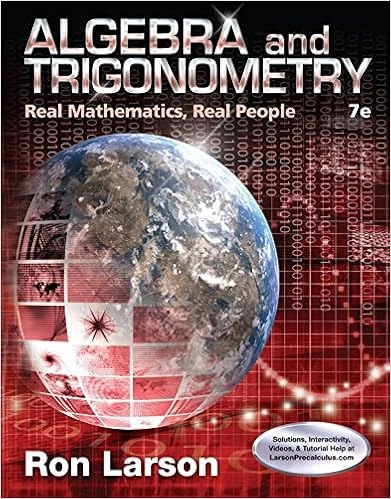# Assume that a bond pays a semi annual coupon of 50

• Test Prep
• 60
• 100% (1) 1 out of 1 people found this document helpful

This preview shows page 7 - 16 out of 60 pages.

##### We have textbook solutions for you!
The document you are viewing contains questions related to this textbook.The document you are viewing contains questions related to this textbook.
Chapter 4 / Exercise 66
Algebra and Trigonometry: Real Mathematics, Real People
LarsonExpert Verified
20. Assume that a bond pays a semi-annual coupon of \$50 and has a current price of \$1,113.14879. Also assume that in one period (six months) the price of this bond will 7
##### We have textbook solutions for you!
The document you are viewing contains questions related to this textbook.The document you are viewing contains questions related to this textbook.
Chapter 4 / Exercise 66
Algebra and Trigonometry: Real Mathematics, Real People
LarsonExpert Verified
Chapter 7 decrease to \$1,111.23682. Given this information, determine the annual yield to maturity for this bond.
Summer 2015 8
Chapter 7 15. Assume that a 10-year bond pays interest of \$36 every six months and will mature for \$1,000. Also assume that the yield to maturity on this bond is currently 7.98 percent. Given this information, determine the current price of this bond.
15. Assume that a 10-year bond pays interest of \$36 every six months and will mature for \$1,000. Also assume that the yield to maturity on this bond is currently 7.98 percent. Given this information, determine the current price of this bond. 9
Chapter 7 *
Duration 10
Chapter 7 9. Assume that you are given the following cash flows for a bond that pays an annual coupon of \$95 and matures for \$1,000. Given this information, determine the duration of this bond if the annual yield-to-maturity is 12.0 percent. Year Cash Flow 1 \$95.00 2 \$95.00 3 \$95.00 4 \$95.00 5 \$1,095.00 A. 4.16 years B. 4.34 years C. 4.51 years D. 4.69 years E. 4.86 years
11
Chapter 7 9. Assume that you are given the following cash flows for a bond that pays an annual coupon of \$95 and matures for \$1,000. Given this information, determine the duration of this bond if the annual yield-to-maturity is 12.0 percent. Year Cash Flow 1 \$95.00 2 \$95.00 3 \$95.00 4 \$95.00 5 \$1,095.00 *
2010 12
Chapter 7 25. Assume that you purchase a 5-year bond that has a par value of \$1,000, annual coupon payments of \$90 each year, and a current price of \$891.86. Based on this information, determine the duration (in years) of this bond if the annual yield-to-maturity is 12.0 percent. A table has been provided to help you organize your calculations. Year Cash Flow Weighted Cash Flow PV of Cash Flow PV of Weighted Cash Flow 1 \$90.00 \$80.36 2 \$90.00 \$71.75 3 \$90.00 \$64.06 4 \$90.00 \$57.20 5 \$1,090.00 \$618.50 Value = \$891.86
25. Assume that you purchase a 5-year bond that has a par value of \$1,000, annual coupon payments of \$90 each year, and a current price of \$891.86. Based on this information, 13
Chapter 7 determine the duration (in years) of this bond if the annual yield-to-maturity is 12.0 percent. A table has been provided to help you organize your calculations. Year Cash Flow Weighted Cash Flow PV of Cash Flow PV of Weighted Cash Flow 1 \$90.00 \$80.36 2 \$90.00 \$71.75 3 \$90.00 \$64.06 4 \$90.00 \$57.20 5 \$1,090.00 \$618.50 Value = \$891.86
Year Cash Flow Weighted Cash Flow PV of Cash Flow PV of Weighted Cash Flow 1 \$90.00 \$90.00 \$80.36 \$80.36 2 \$90.00 \$180.00 \$71.75 \$143.49 3 \$90.00 \$270.00 \$64.06 \$192.18 4 \$90.00 \$360.00 \$57.20 \$228.79 5 \$1,090.00 \$5,450.00 \$618.50 \$3,092.48 Value = \$891.86 \$3,737.30 Duration = \$3,737.30 / \$891.86 = 4.19 2012 14
Chapter 7
•••